# Test: LCM & GCD - 3

## 15 Questions MCQ Test Quantitative Reasoning for GMAT | Test: LCM & GCD - 3

Description
Attempt Test: LCM & GCD - 3 | 15 questions in 30 minutes | Mock test for GMAT preparation | Free important questions MCQ to study Quantitative Reasoning for GMAT for GMAT Exam | Download free PDF with solutions
QUESTION: 1

### What is HCF of  36/75, 48/150 , 72/135?

Solution: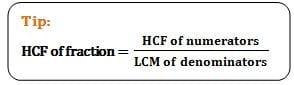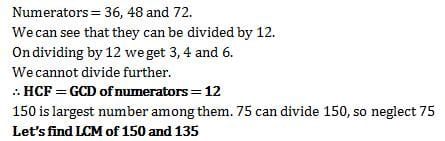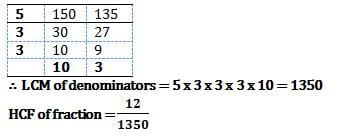QUESTION: 2

### Rajesh had to arrange his books in uniform groups. He makes groups of 4 books each. But 3 books are left. He tries it with groups of 5 books each. But still 3 books are left. 3 books are still left when he tried with groups of 9 or 10 books each. How many books does he have?

Solution:

Since we need total number of books, we must find LCM of 4,5,9,10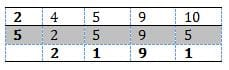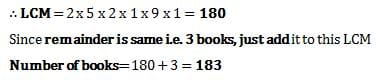QUESTION: 3

### HCF and LCM of two numbers is 8 and 96. Sum of those numbers is 56. Then what is sum of their reciprocals?

Solution: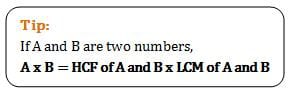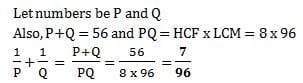QUESTION: 4

What is LCM of  36/225, 48/150 , 72/65 ?

Solution: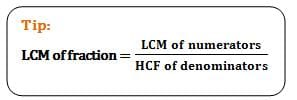Numerators = 36, 48 and 72.
72 is largest number among them. 72 is not divisible by 36 or 48
72 x 2 = 144 = divisible by 72, 36 and 48
∴ LCM of numerators =144
Denominators= 225, 150 and 65.
We can see that they can be divided by 5.
On dividing by 5 Imeget 45, 30 and 13
We cannot divide further. So, HCF = GCD =5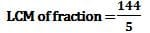QUESTION: 5

Manoj wants to paste wallpaper on wall of his room. The wall is 4 meters and 50 cm in length and 3 meters and 50 cm in height. But the wall should be covered completely only by square pieces of wallpaper having same size. What is the number of maximum sized wallpaper squares needed to cover the walls completely?

Solution:

Wall can be covered only by using square sized wallpaper pieces.
Different sized squares are not allowed.
Length = 4 m 50 cm = 450 cm ; Height = 3 m 50 cm = 350 cm
Maximum square size of possible means HCF of 350 and 450
We can see that 350 and 450 can be divided by 50.
On dividing by 50, we get 7 and 9.
Since we cannot divide further, HCF = 50 = size of side of square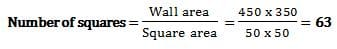QUESTION: 6

The ratio of two numbers is 5 : 6 and their LCM is 480, then their HCF is?

Solution: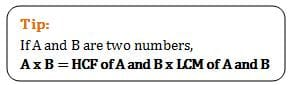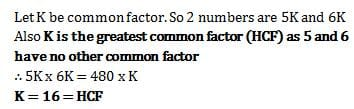QUESTION: 7

The length and breadth of a rectangular floor are 16.25 metre and 12.75 metre respectively. Find how many minimum number of square tiles would be required to cover it completely?

Solution:

Since we require minimum number of square tiles, the size of the tile is given as the H.C.F. of two sides of the room. The H.C.F. Of 1625 cm & 1275 cm. is 25 cms. Hence, we get,
Required Number = (1625 * 1275) / (25 * 25) = 3315

QUESTION: 8

The L.C.M. of two number is 60. The numbers are in the ratio 4 : 5. Find the sum of numbers.

Solution:

Let the numbers be 4x and 5x.
Then, their L.C.M. = 20x.
20x = 60 or x = 3.
The numbers are 12 and 15.
Hence, required sum = (12 + 15) = 27.

QUESTION: 9

The L.C.M. Of 2, 2.7 and 0.09 is:

Solution:

Given numbers are 2.00, 2.70 and 0.09.
L.C.M. of 200, 270 and 9 is 5400.
L.C.M. Of given numbers = 54.00 = 54

QUESTION: 10

Find the least number which should be added to 2430 so that the sum is exactly divisible by 5, 4 and 2

Solution:

L.C.M of 5, 4, and 2 = 20. On dividing 2430 by 20, the remainder is 10.
Therefore, number to be added = (20 - 10) = 10

QUESTION: 11

The ratio of two numbers is 4 : 5 and their H.C.F is 4. Find their L.C.M.

Solution:

Let the numbers be 4x and 5x.

H.C.F = x. So, x=4.
So, the numbers are 16 and 20
L.C.M of 16 and 20 = 80.

QUESTION: 12

A man decides to pave with square tiles his hall which is 4.8 metres long and 5.38 metres wide. Find the largest size of the tile that he could use.

Solution:

The largest size of the tile is H.C.F. of 480 cm and 538 cm is 2 cm.

QUESTION: 13

3 bells beep at an interval of 12, 20, and 35 minutes. If they beep together at 10 a.m., then they will again beep together at:

Solution:

The L.C.M. of 12, 20 and 35 is 420. Hence, all 3 bells beep together after 420 minutes = 7 hours
Hence, the 3 bells will beep together 7 hours after 10 a.m. i.e. at 5 p.m.

QUESTION: 14

The least number which is a multiple of 11 and when divided by 3, 5 and 9 leaves 2 as remainder:

Solution:

The L.C.M. of 3, 5 and 9 is 45.
Hence, the number is of the form (45a + 2).
The least value of ‘a’ for which (45a + 2) is multiple of 11 is 9.
Therefore, the number is 407.

QUESTION: 15

LCM of two numbers is 495. HCF of these numbers is 5. If the sum of the numbers is 100, find out the difference between the two numbers.

Solution:

It is given that sum of two numbers is 100. Let the two numbers be x and 100 – x and HCF is 5 and LCM is 495

=> We need to apply the formula: HCF x LCM = Product of two numbers

Which gives x(100 – x) = 495 x 5

Solving the equation we get a quadratic which looks like this: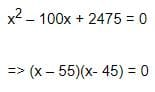Therefore, Two numbers are 55 and 45Use Code STAYHOME200 and get INR 200 additional OFF Use Coupon Code